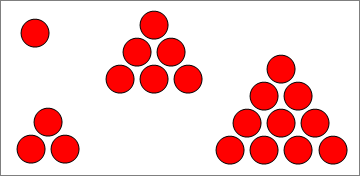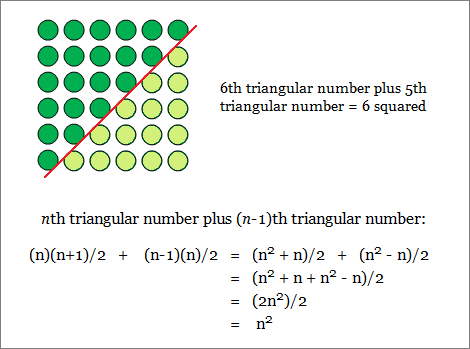# Sum of Consecutive Integers and Triangular Numbers Calculator

Sum Calculator
Find the sum of consecutive integers from
to inclusive

The sum of the first n integers, 1 + 2 + 3 + ... + n, is called the nth triangular number. Triangular numbers are so-called because they can be represented by triangular formations.Triangular numbers and the sum of any sequence of consecutive integers can either be calculated the long way by adding each term individually, or the short way by using a simple formula.

### Triangular Number Formula

Let T(n) be the nth triangular number. We know that

T(n) = 1 + 2 + ... + (n-1) + n.       n terms

Similarly,

2*T(n) = 1 + 2 + ... + (n-1) + n + 1 + 2 + ... + (n-1) + n.

We can rearrange the terms of 2*T(n) by pairing the smallest term with the largest term, the second smallest term with the second largest term, etc. This new arrangement doesn't change the value of the sum; it does provide an easier way to calculate its value:

2*T(n) = [1 + n] + [2 + (n-1)] + ... + [(n-1) + 2] + [n + 1]       n terms
= [1 + n] + [1 + n] + ... + [1 + n] + [1 + n]
= n(n+1).

Thus, T(n) = n(n+1)/2.

### Consecutive Integer Sum Formula

The sum of consecutive numbers from a to b inclusive is the difference between the bth triangular number and the ath triangular number plus a. (You need to add a because the sum is inclusive of the endpoints a and b.) That is,

Sum from a to b inclusive = T(b) - T(a) + a.

Equivalently:

T(b) - T(a) + a = (b+a)(b-a+1)/2.

### Fun Facts about Triangular Numbers

1. The sum of two consecutive triangular numbers is always square. This is best explained by the picture below:2. There are infinitely many triangular numbers that are also square numbers, that is, T(x) = y² for suitable values of x and y. The table below shows the first six sets:
 x(x+1)/2 = y2 x y 1 1 8 6 49 35 288 204 1681 1189 9800 6930
If X(n) is the nth entry in the x column and Y(n) is the nth entry in the y column, then X(n) and Y(n) satisfy the recursive relations

X(n+2) = 6X(n+1) - X(n) + 2
Y(n+2) = 6Y(n+1) - Y(n).

Also, X(n) is itself a square number for odd values of n, and twice a square number for even values of n.

3. The sum of the first n triangular numbers is the nth tetrahedral (triangular pyramidal) number, P(n).

T(1) + T(2) + ... + T(n) = P(n), and

P(n) = n(n+1)(n+2)/6.

4. The infinite sum of the reciprocals of the triangular numbers is 2. This can be demonstrated as follows:

Σn=1...∞1/T(n) = Σn=1...∞2/[n(n+1)]

= 2Σn=1...∞[1/n - 1/(n+1)]

This is a telescoping sum, meaning consecutive terms in the series cancel out, which leaves only the first term, 1. Thus, the sum is equal to 2(1) = 2.